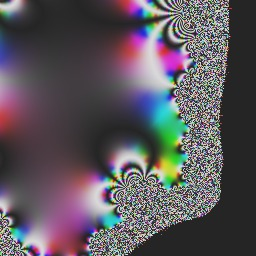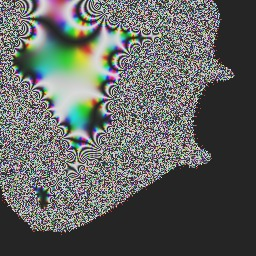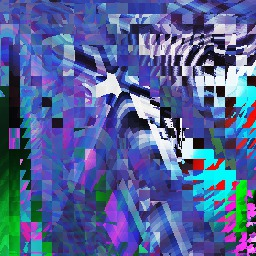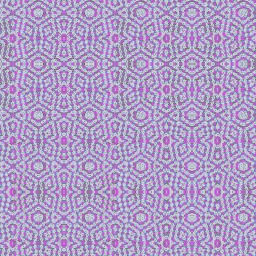# Random art

The pictures in the gallery are made by a computer program. The program accepts the name of a picture and uses it as a seed from which a picture is generated randomly. The same name always yields the same picture.

You can make your own picture, too.

The author of the random art program is Andrej Bauer.

### How it works

The picture name is the seed for a pseudo-random number generator that is used to construct a mathematical formula. The formula determines the color of each pixel in the picture. The same name always determines the same sequence of random choices, and consequently the same formula and the same picture.

A typical formula describing a picture looks like this:

[|palette_pp(3,1); pif(9,2,4); pclosestmax(13,16); protfold(5,13); pif(41,10,7); pfoci(27,6,40); pfoci(23,8,32); pplus(11,8); fold(11,23,16); and(15,12); protfold(13,29); rotate(28,27,17); fless(18,16); pif(24,14,14); pmult(28,23); negative(20); fclosest(18,20); fplus(19,25); fplus(20,25); max(22,22); fclosest(34,21); dist(23,23); fclosest(45,25); fold(27,46,54); and(51,41); fmix(45,33,26); fplus(45,36); fold(30,28,34); fold(40,30,38); pclosestmax(49,38); fold(31,32,35); pmix(39,37,36); pmix(44,55,35); dist(58,46); fplus(61,38); dist(39,43); fmix(38,38,38); rotate(58,43,38); fmix(45,61,45); fold(40,43,61); rotate(42,42,61); or(47,47); pif(47,46,48); pmult(58,60); torus(48,45,61); fclosest(61,61); pif(47,58,48); negative(61); torus(59,61,61); fold(55,50,54); discretize(55,56); or(52,52); fless(54,53); dist(56,60); fclosest(61,61); pif(57,56,56); pclosestmax(60,61); negative(61); torus(60,61,61); pfoci(60,60,60); pt(); t()|]

It is a list of operators, which refer to other operators in the list. They comprise an acyclic directed graph. It is usually quite impossible to predict the appearance of a picture just by looking at the formula.

It may happen that two different names determine the same picture, although this is rather unlikely due to the large number of all possible pictures. It is hard to determine their precise number, but we may safely claim that there are more random pictures than there are atoms in the known universe.

The name of a picture normally consists of two words. The first word determines the colors and the layout of focal points, while the second word determines the selection and arrangement of graphical elements. Therefore, if we change the first word and keep the second, the colors and the layout change while the style remains the same:Red DemoGreen DemoBlue Demo

In contrast, if we keep the first word and change the second one, we obtain different pictures whith few similarities:Demo RedDemo GreenDemo Blue

The program is implemented in ocaml. The online version was compiled from ocaml to javascript with ocamljs. The program is not open source but you may download the publicly available Python implementation if you would like to experiment with computer art.

For further information and discussion about random art visit random art at the Mathematics and Computation blog or join the Facbook Random Art group.